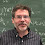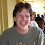## Tuesday, January 17, 2012

### How important are the Fib numbers in math? in Nature? In History of Math books?

The following quotes is from In the book Algebra in Ancient and Modern Times by V.S. Varadarajan.
Fibonacci numbers thus grow very fast with N, indeed in geometric progression. This is often called exponential growth. They remained as curiosities till in the 1960's they were found to be crucial in certain studies in mathematical logic.
I suspected they were refering to its use in Hilbert's tenth problem even though that was really 1970 (a quibble) and I would hardly call it crucial (a more substantial objection). In fact Fib Numbers are not even needed in the end. I asked Chris Lastowksi who is a Model Theorist at UMCP and he told me the folowing:
Yes. Matijasec showed that the Fibonacci sequence was diophantine, and this sufficed to solve Hilbert's tenth problem (actually to show it could not be solved), by earlier work of Davis, J. Robinson and Putnam. However, Davis almost immediately showed that the exponential function is diophantine, which yields the solution to H-10 more easily, so I would hardly call that a deep connection.
V.S. Varadarajan wanted to make the Fib numbers interesting and important. The attempt was not quite right.
1. How bad is it for a history-of-math book to exaggerate how important some concept is?
2. How important are the Fib Numbers? Do they come up in Mathematics?
3. Could V.S. Varadarajan have picked a better example of their use in mathematics?
4. It has been said that the Fib Numbers come up in Nature. According to Fib Flim Flam most of the statements made about Fib numbers and nature are suspect.

1.How about the role of the Fibonacci numbers as the worst-case of Euclid's algorithm for the GCD? That seems pretty fundamental.

2.Related to last Shallit's comment: Fibonacci words are often used as worst-case examples in stringology. Altough this does not mean that we could not live without them.

3.In Computer Science we have Fibonacci Heaps.

4.In my opinion, one of the biggest contributions Fibonacci numbers make to math (that isn't too obscure) is a contribution which doesn't get **anywhere near** as much attention as it deserves. Namely, they contribute a neat application example to matrix diagonalization (an otherwise dry subject which the Fibonacci example really breathes life into). What on Earth am I talking about? Once you know how to diagonalize matrices, it becomes routine to derive the formula for the nth Fibonacci number, a formula which is otherwise somewhat mystical-seeming. (For details see: http://mathproofs.blogspot.com/2005/04/nth-term-of-fibonacci-sequence.html )

Is Matijasec some weird alternate spelling? I've seen Matiyasevich spelled several different ways but never THAT way...

5.1) In the above examples do you really need FIB
(1,1,2,3,5,8,...) or would any sequence satisfying
a(n)=a(n-1)+a(n-2),perhaps with diff initial conditions, suffice.

Matijasec Hilbert

the first hit you get is... This blog. I am sure that the way I
spelled it is not an alternative spelling but is just plain wrong.
(Will fix later.)

6.I think you meant Matijasevic.

7.Yet further to Jeffrey Shallit's comment, Fibonacci numbers are also the worst case in Huffman coding. They also turn out to be "harmonics" in many algorithms, to the extent that if you're graphing the performance of certain algorithms for problems of various size, Fibonacci numbers are often outliers. (That, or powers of two.)

As well as Fibonacci heaps, we have Fibonacci codes and a bunch of other constructions which rely on Fibonacci numbers.

So at the very least, Fibonacci numbers are a useful design and analysis tool in disparate areas of computer science.

8.In my experience, the Fibonacci numbers are an incredibly valuable tool in mathematics. I can say with near certainty that the most important application of Fibonacci numbers is as a toy. There are any number of compelling results about them which become a gateway for learning induction, modular arithmetic, and a passion for the beauty of mathematics. I would think that countless mathematicians were set on that path with the help of Fibonacci numbers, thus leading to countless interesting theorems having nothing to do with them.

There seem to be other interesting applications listed above, but none are as important as the role of attraction.

9.I think the official statement to be made against the author in these times is "citation needed".